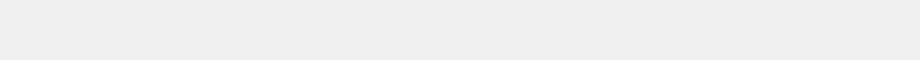API Details
Tutorial Examples
NousMind, NousCrypt, and NousRandom are trademarks of Scott Michael Doctor
Patents Pending
The following examples provide a quick primer for using the API. Each command shown is described in detail in the relevant section of this reference maual.

Example 1:     We want 10 numbers.

https://nousrandom.net/api/v1/?cnt=10

Note the question mark. The question mark must be the first character of the query string. the cnt command is the count of numbers to be returned, in this example being set to 10. If you copy the URL into your web browser you will get 10 numbers returned.

You can append the debug command to that URL string as:

https://nousrandom.net/api/v1/?cnt=10;debug

which will return the API interpretation of the query:

https://nousrandom.net/api/v1/?flt;min=-1.0;max=1.0;cnt=10;scioff

Each time you refresh, or submit with a GET the query string, a new set of 10 numbers will be returned by the API.

Example 2:     We want 10 numbers arranged in two columns of 5 numbers.

https://nousrandom.net/api/v1/?cnt=10;cols=2

Note the semicolon separating the two commands. Do not use any spaces in the query string. The cols command specifies how many columns to use when returning the numbers. The example will return two columns separated by a single space character with 5 numbers in each column.

Example 3:     10 numbers, 2 columns, but we want the numbers scaled from 1.0 to 20.0

https://nousrandom.net/api/v1/?cnt=10;cols=2;min=1.0;max=20.0

The min and max commands set the minimum and maximum values. Since the internal numbers are normalized [-1.0,1.0], scaling involves a multiplication of the differential magnitude then adding an offset. This is all handled by the API internally by setting the max and min values. Any real numbers may be used for these values. The returned numbers from this example will have floating point values between 1.0 and 20.0 inclusive. The example shows the values with a decimal, however specifying as min=1;max=20 will return the same result in floating point. I used the decimal to show you can use fractional numbers. A fractional number less than 1.0 must have a leading zero such as 0.25. numbers may also be specified in scientific notation.

Example 4:      10 numbers, 2 columns, scaled [1.0,20.0], but we want integers.

https://nousrandom.net/api/v1/?int;cnt=10;cols=2;min=1;max=20

The int command is a unary operator that tells the API to return just the integer value of the numbers. A min and max value must be specified when using int. Only the integer value of min and max is used. any fractional part is discarded. The difference between min and max must be at least 1.0.

Note that the API uses a custom rounding algorithm to ensure a uniform distribution of numbers, especially with regard to the boundary numbers. The reference page about Random Integers explains the algorithm.

Example 5:     20 integer numbers, but we want them all unique.

https://nousrandom.net/api/v1/?uniqint;min=1;max=20

A unique list is a draw without replacement, such as drawing numbers out of a hat, lottery numbers, and such. Each number is drawn exactly once. The uniqint command tells the API to use a scrambling algorithm to select the numbers. The algorithm is explained in my theory paper.

Each number is selected exactly once in a random order. Note that the length of a unique integer list is defined by the min and max command. Internally the cnt command is set to the total possible numbers which overrides any setting of the cnt command, so the cnt command is not used here. Specifying a value for cnt has no effect when using the uniqint command.

Another option is the uniqflt command which returns a list of equally spaced floating point numbers. See the reference page about Unique Numbers for details.

Example 6:     20 unique integers, but we only want to draw 3 numbers from the set.

https://nousrandom.net/api/v1/?uniqint;min=1;max=20;draw=3

The draw command specifies how many numbers to draw from the set of unique numbers. For example three winners out of 20 entries. The draw command is only used with the uniqint command. it has no effect without the uniqint command. Without specifying draw, the entire set will be returned.